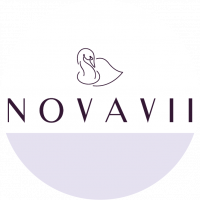# What Is Return on Investment (ROI)?Selected

#### Novavii

1 year ago
Let's say your total investment is X.
The Amount your investment increases to become is Y.

Then, ROI = (Y-X)/X * 100
eg:)
If total investment X = Rs. 1000
If this investment increases to Y = Rs. 2000
Then ROI (Y-X)/X = (2000-1000)/1000 * 100
= 100%
= Rs. 1000#### Krishna Rath

1 year ago
If₹100 is invested and you get ₹120 after a year, then RoI (return on investment) is ₹20 or 20%.